Скачать презентацию GPLAG Detection of Software Plagiarism by Program Dependence

cd24de6abe63551e30a63e0b8f8b4a4a.ppt

• Количество слайдов: 42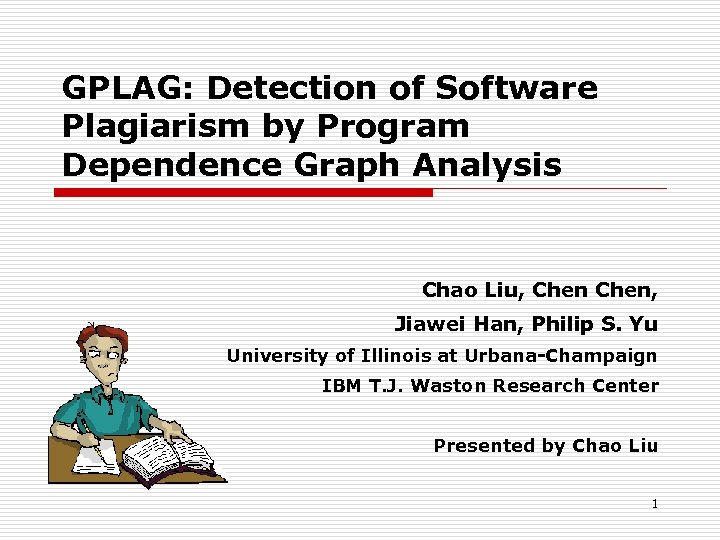GPLAG: Detection of Software Plagiarism by Program Dependence Graph Analysis Chao Liu, Chen, Jiawei Han, Philip S. Yu University of Illinois at Urbana-Champaign IBM T. J. Waston Research Center Presented by Chao Liu 1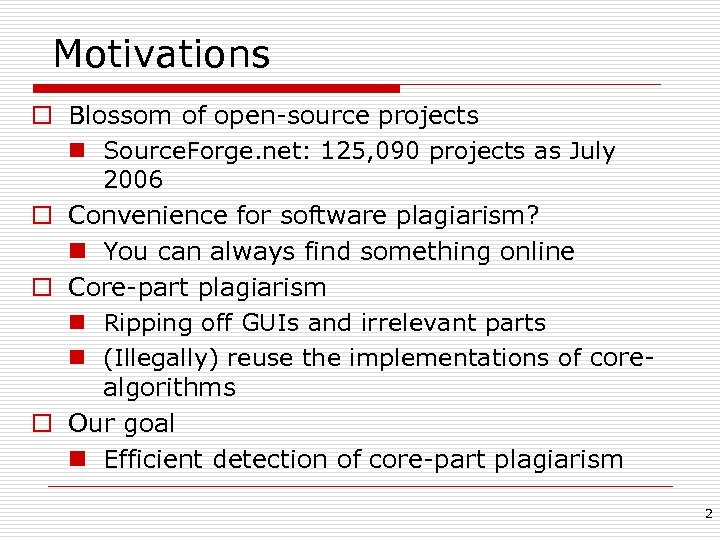Motivations o Blossom of open-source projects n Source. Forge. net: 125, 090 projects as July 2006 o Convenience for software plagiarism? n You can always find something online o Core-part plagiarism n Ripping off GUIs and irrelevant parts n (Illegally) reuse the implementations of corealgorithms o Our goal n Efficient detection of core-part plagiarism 2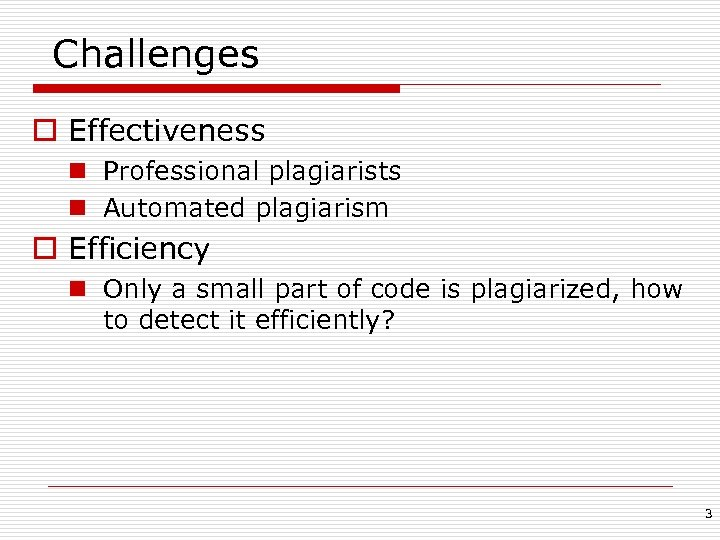Challenges o Effectiveness n Professional plagiarists n Automated plagiarism o Efficiency n Only a small part of code is plagiarized, how to detect it efficiently? 3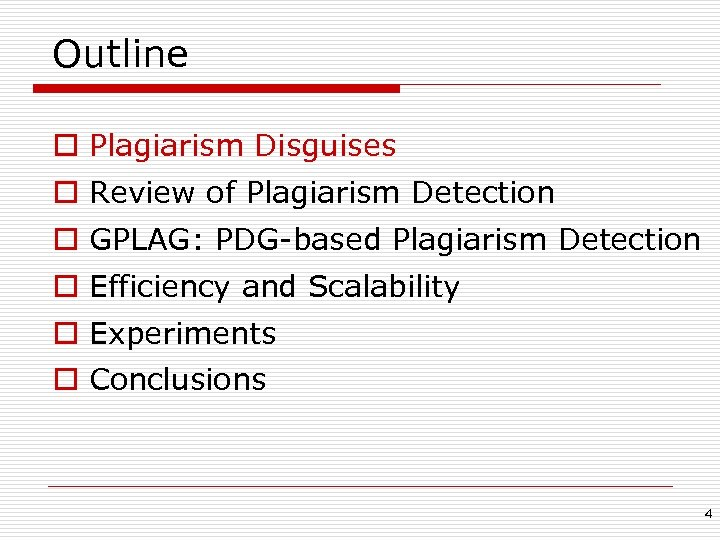Outline o Plagiarism Disguises o Review of Plagiarism Detection o GPLAG: PDG-based Plagiarism Detection o Efficiency and Scalability o Experiments o Conclusions 4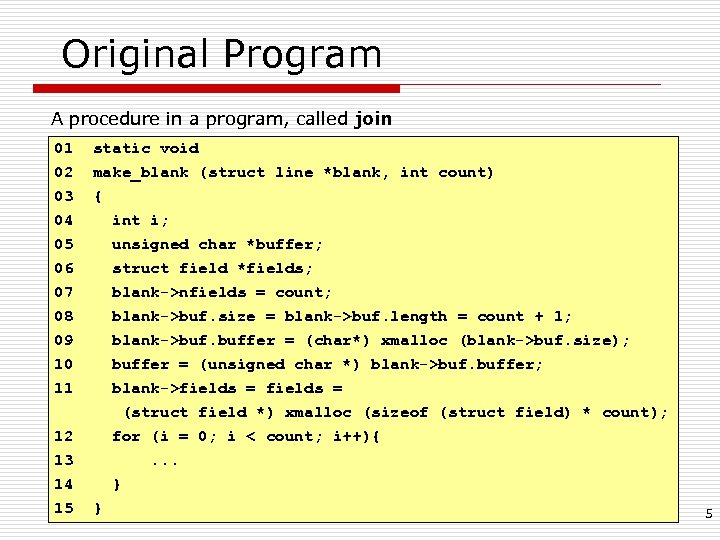Original Program A procedure in a program, called join 01 static void 02 03 make_blank (struct line *blank, int count) { 04 05 06 07 08 09 10 11 12 13 14 15 int i; unsigned char *buffer; struct field *fields; blank->nfields = count; blank->buf. size = blank->buf. length = count + 1; blank->buf. buffer = (char*) xmalloc (blank->buf. size); buffer = (unsigned char *) blank->buf. buffer; blank->fields = (struct field *) xmalloc (sizeof (struct field) * count); for (i = 0; i < count; i++){. . . } } 5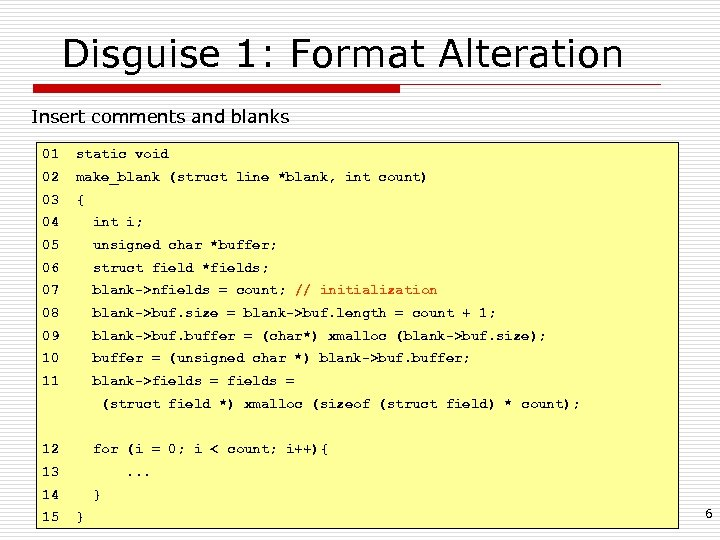Disguise 1: Format Alteration Insert comments and blanks 01 static void 02 make_blank (struct line *blank, int count) 03 { 04 int i; 05 unsigned char *buffer; 06 struct field *fields; 07 blank->nfields = count; // initialization 08 blank->buf. size = blank->buf. length = count + 1; 09 blank->buf. buffer = (char*) xmalloc (blank->buf. size); 10 buffer = (unsigned char *) blank->buf. buffer; 11 blank->fields = (struct field *) xmalloc (sizeof (struct field) * count); 12 for (i = 0; i < count; i++){ 13 . . . 14 15 } } 6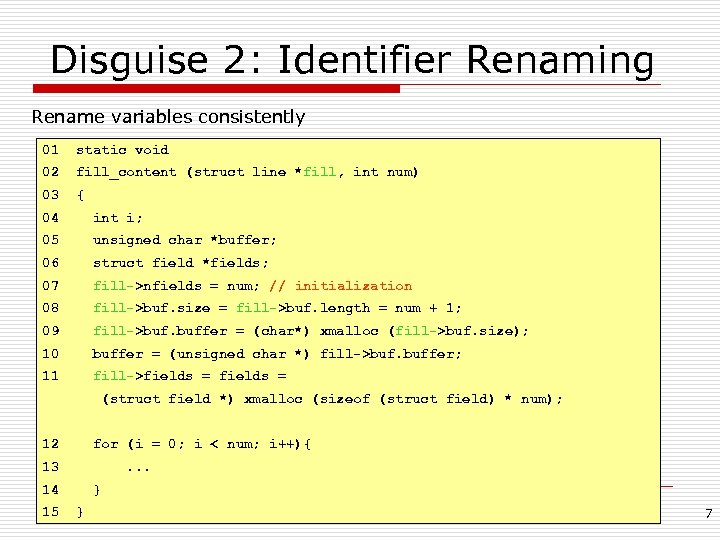Disguise 2: Identifier Renaming Rename variables consistently 01 static void 02 fill_content (struct line *fill, int num) 03 { 04 int i; 05 unsigned char *buffer; 06 struct field *fields; 07 fill->nfields = num; // initialization 08 fill->buf. size = fill->buf. length = num + 1; 09 fill->buf. buffer = (char*) xmalloc (fill->buf. size); 10 buffer = (unsigned char *) fill->buf. buffer; 11 fill->fields = (struct field *) xmalloc (sizeof (struct field) * num); 12 for (i = 0; i < num; i++){ 13 . . . 14 15 } } 7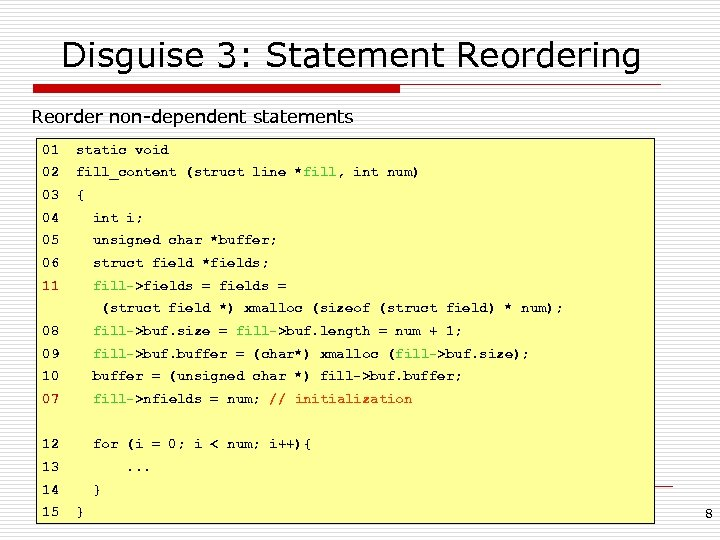Disguise 3: Statement Reordering Reorder non-dependent statements 01 static void 02 fill_content (struct line *fill, int num) 03 { 04 int i; 05 unsigned char *buffer; 06 struct field *fields; 11 fill->fields = (struct field *) xmalloc (sizeof (struct field) * num); 08 fill->buf. size = fill->buf. length = num + 1; 09 fill->buf. buffer = (char*) xmalloc (fill->buf. size); 10 buffer = (unsigned char *) fill->buf. buffer; 07 fill->nfields = num; // initialization 12 for (i = 0; i < num; i++){ 13 . . . 14 15 } } 8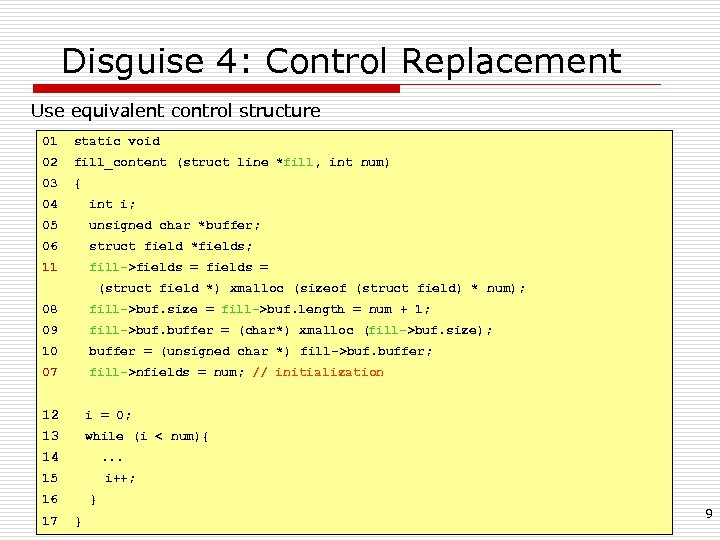Disguise 4: Control Replacement Use equivalent control structure 01 static void 02 fill_content (struct line *fill, int num) 03 { 04 int i; 05 unsigned char *buffer; 06 struct field *fields; 11 fill->fields = (struct field *) xmalloc (sizeof (struct field) * num); 08 fill->buf. size = fill->buf. length = num + 1; 09 fill->buf. buffer = (char*) xmalloc (fill->buf. size); 10 buffer = (unsigned char *) fill->buf. buffer; 07 fill->nfields = num; // initialization 12 i = 0; 13 while (i < num){ 14 . . . 15 i++; 16 17 } } 9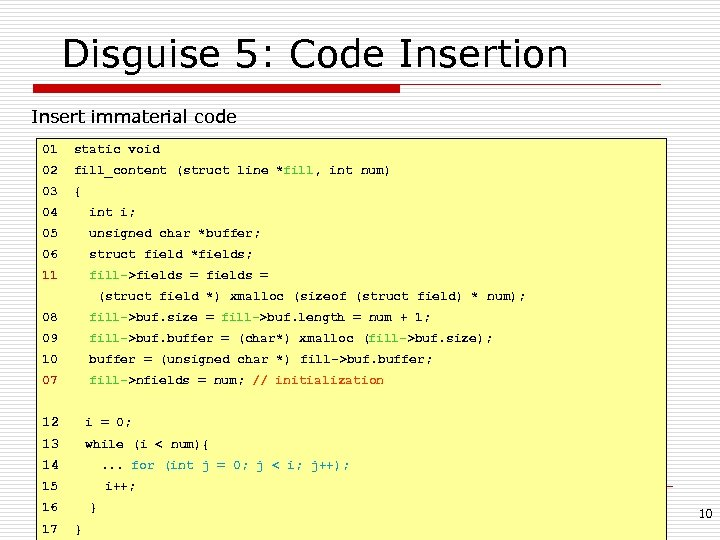Disguise 5: Code Insertion Insert immaterial code 01 static void 02 fill_content (struct line *fill, int num) 03 { 04 int i; 05 unsigned char *buffer; 06 struct field *fields; 11 fill->fields = (struct field *) xmalloc (sizeof (struct field) * num); 08 fill->buf. size = fill->buf. length = num + 1; 09 fill->buf. buffer = (char*) xmalloc (fill->buf. size); 10 buffer = (unsigned char *) fill->buf. buffer; 07 fill->nfields = num; // initialization 12 i = 0; 13 while (i < num){ 14 . . . for (int j = 0; j < i; j++); 15 i++; 16 17 } } 10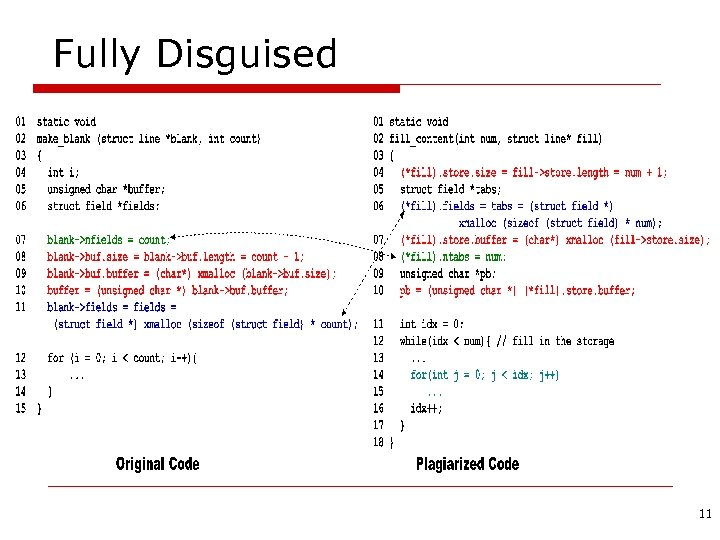Fully Disguised 11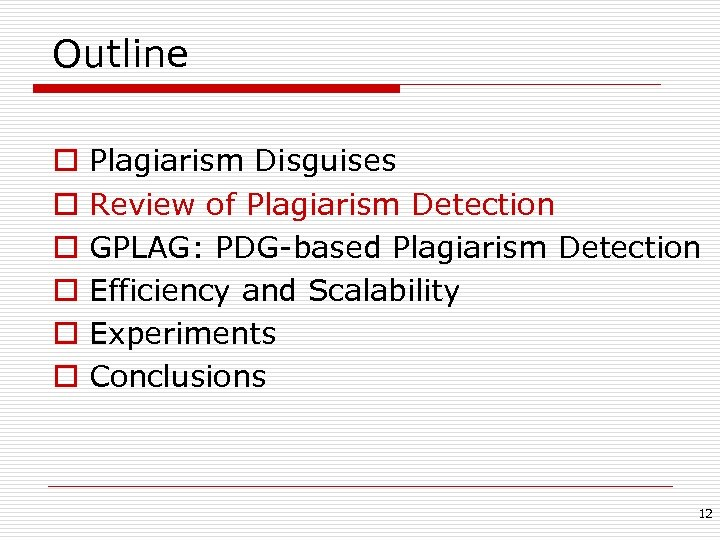Outline o o o Plagiarism Disguises Review of Plagiarism Detection GPLAG: PDG-based Plagiarism Detection Efficiency and Scalability Experiments Conclusions 12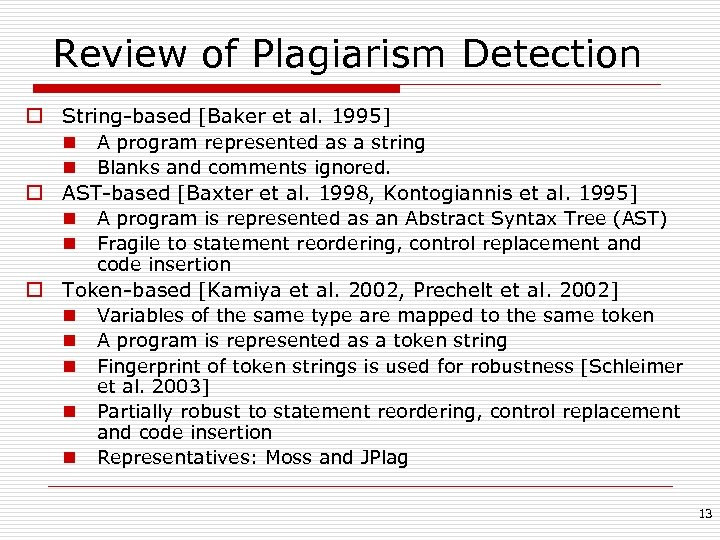Review of Plagiarism Detection o String-based [Baker et al. 1995] n A program represented as a string n Blanks and comments ignored. o AST-based [Baxter et al. 1998, Kontogiannis et al. 1995] n A program is represented as an Abstract Syntax Tree (AST) n Fragile to statement reordering, control replacement and code insertion o Token-based [Kamiya et al. 2002, Prechelt et al. 2002] n Variables of the same type are mapped to the same token n A program is represented as a token string n Fingerprint of token strings is used for robustness [Schleimer n n et al. 2003] Partially robust to statement reordering, control replacement and code insertion Representatives: Moss and JPlag 13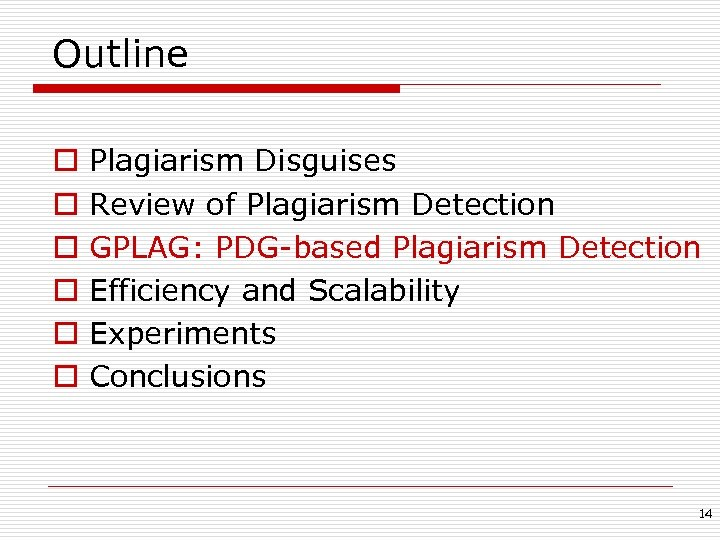Outline o o o Plagiarism Disguises Review of Plagiarism Detection GPLAG: PDG-based Plagiarism Detection Efficiency and Scalability Experiments Conclusions 14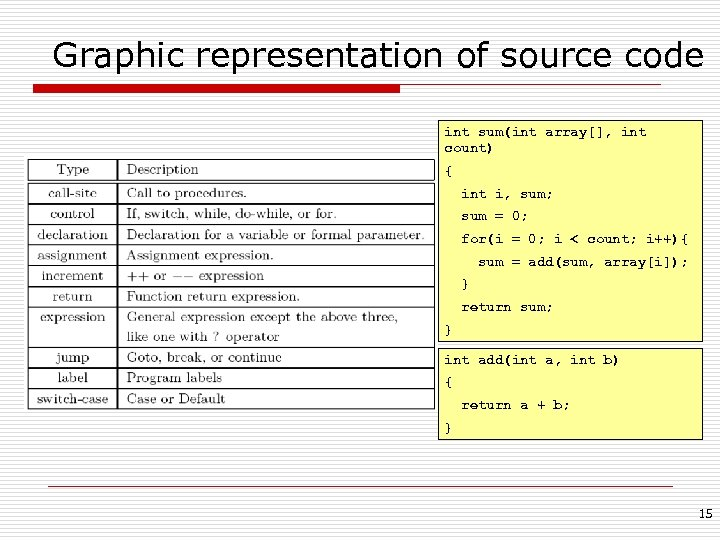Graphic representation of source code int sum(int array[], int count) { int i, sum; sum = 0; for(i = 0; i < count; i++){ sum = add(sum, array[i]); } return sum; } int add(int a, int b) { return a + b; } 15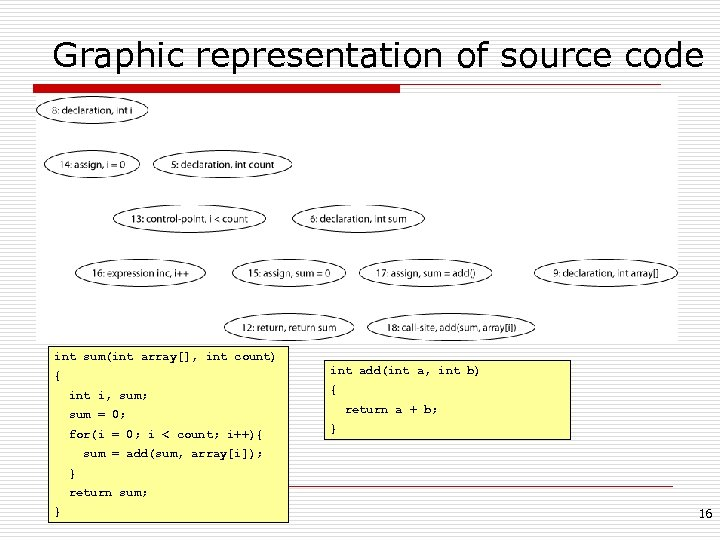Graphic representation of source code int sum(int array[], int count) int add(int a, int b) { int i, sum; { return a + b; sum = 0; for(i = 0; i < count; i++){ } sum = add(sum, array[i]); } return sum; } 16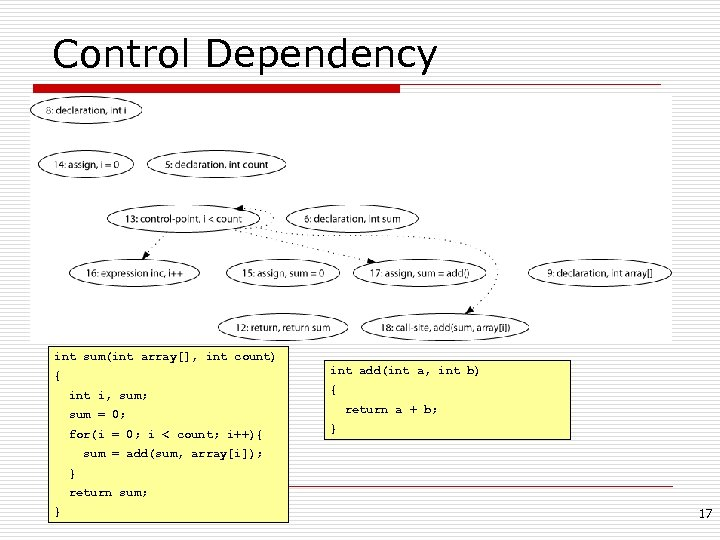Control Dependency int sum(int array[], int count) int add(int a, int b) { int i, sum; { return a + b; sum = 0; for(i = 0; i < count; i++){ } sum = add(sum, array[i]); } return sum; } 17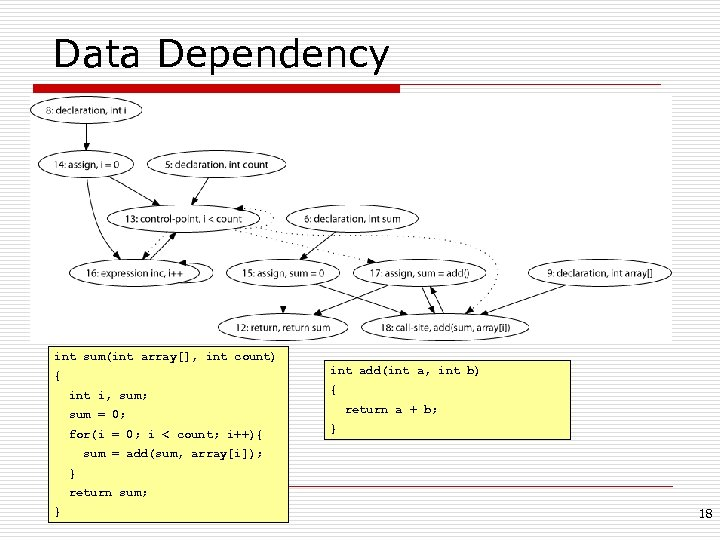Data Dependency int sum(int array[], int count) int add(int a, int b) { int i, sum; { return a + b; sum = 0; for(i = 0; i < count; i++){ } sum = add(sum, array[i]); } return sum; } 18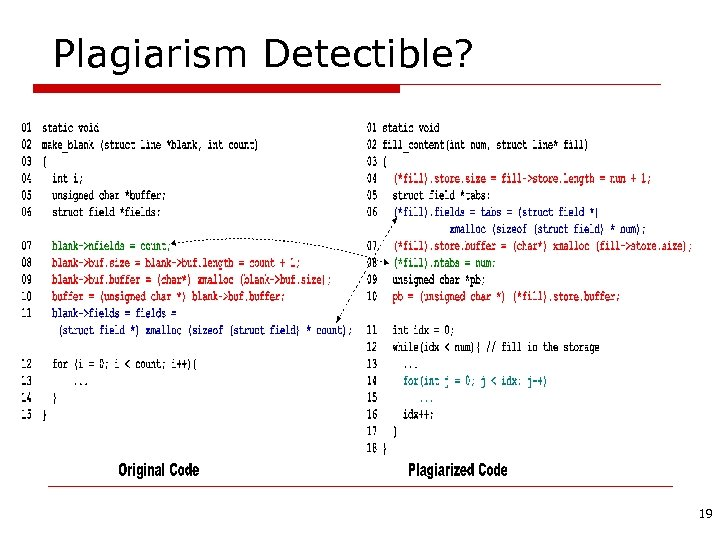Plagiarism Detectible? 19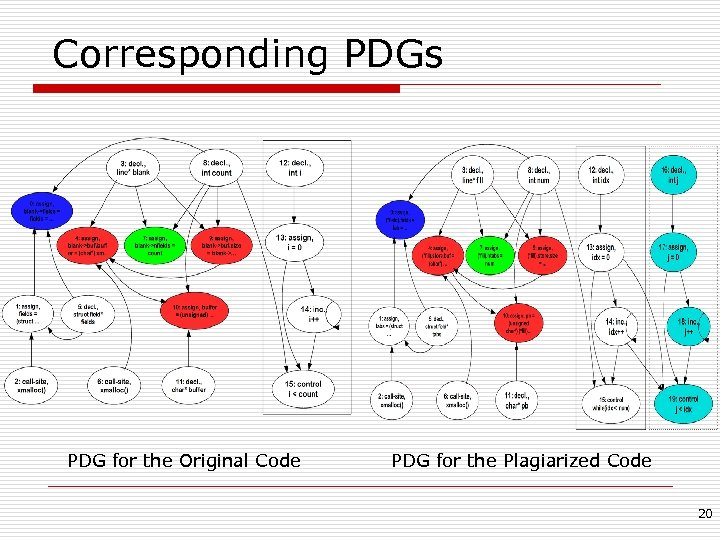Corresponding PDGs PDG for the Original Code PDG for the Plagiarized Code 20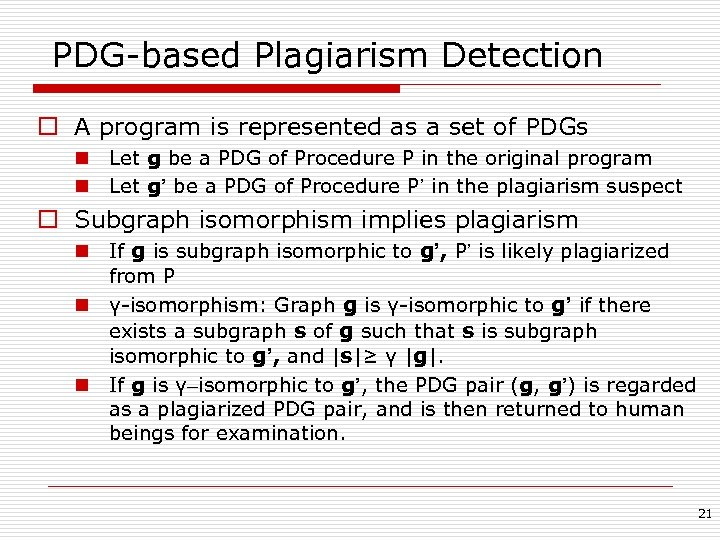PDG-based Plagiarism Detection o A program is represented as a set of PDGs n Let g be a PDG of Procedure P in the original program n Let g’ be a PDG of Procedure P’ in the plagiarism suspect o Subgraph isomorphism implies plagiarism n If g is subgraph isomorphic to g’, P’ is likely plagiarized from P n γ-isomorphism: Graph g is γ-isomorphic to g’ if there exists a subgraph s of g such that s is subgraph isomorphic to g’, and |s|≥ γ |g|. n If g is γ–isomorphic to g’, the PDG pair (g, g’) is regarded as a plagiarized PDG pair, and is then returned to human beings for examination. 21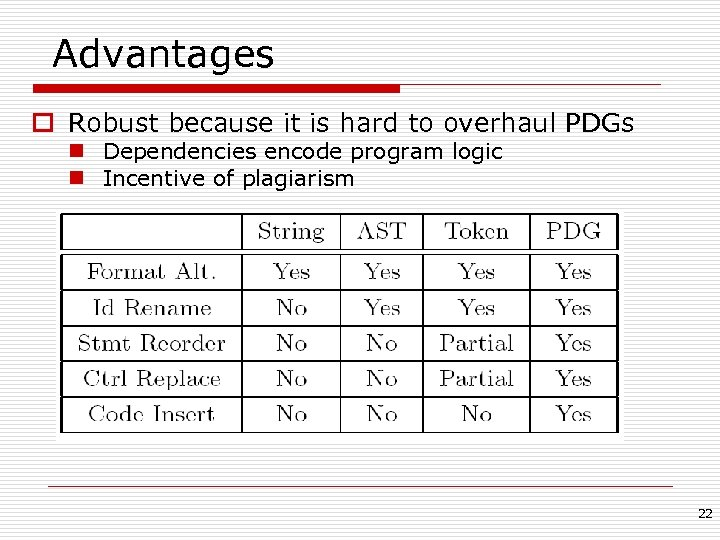Advantages o Robust because it is hard to overhaul PDGs n Dependencies encode program logic n Incentive of plagiarism 22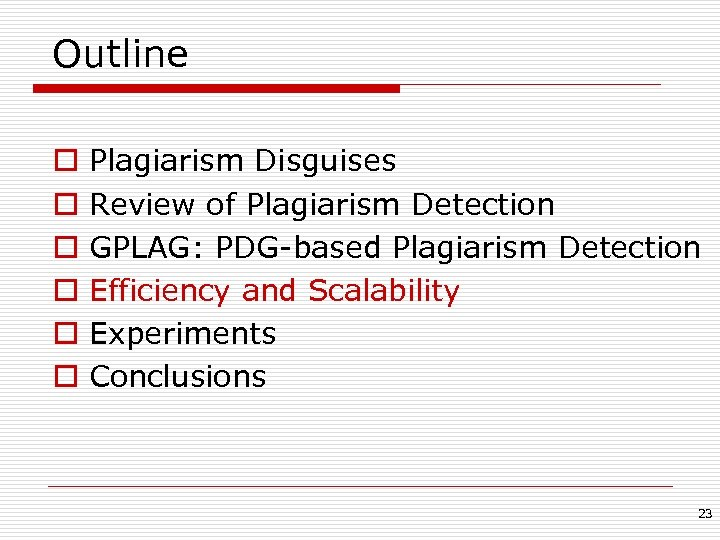Outline o o o Plagiarism Disguises Review of Plagiarism Detection GPLAG: PDG-based Plagiarism Detection Efficiency and Scalability Experiments Conclusions 23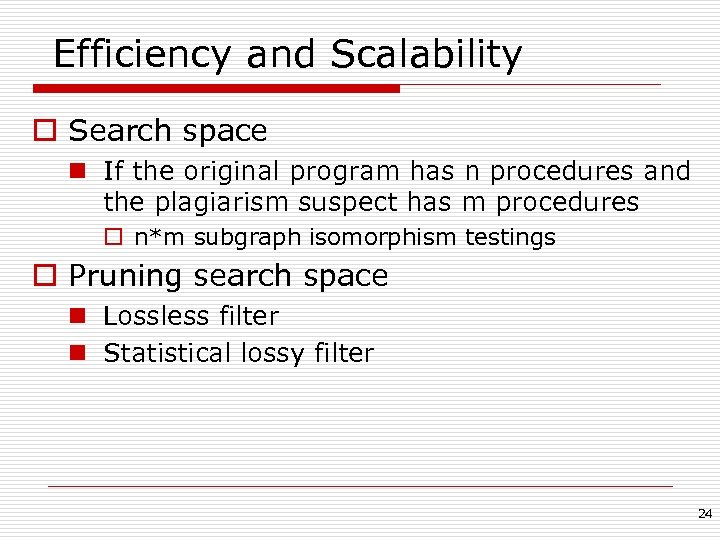Efficiency and Scalability o Search space n If the original program has n procedures and the plagiarism suspect has m procedures o n*m subgraph isomorphism testings o Pruning search space n Lossless filter n Statistical lossy filter 24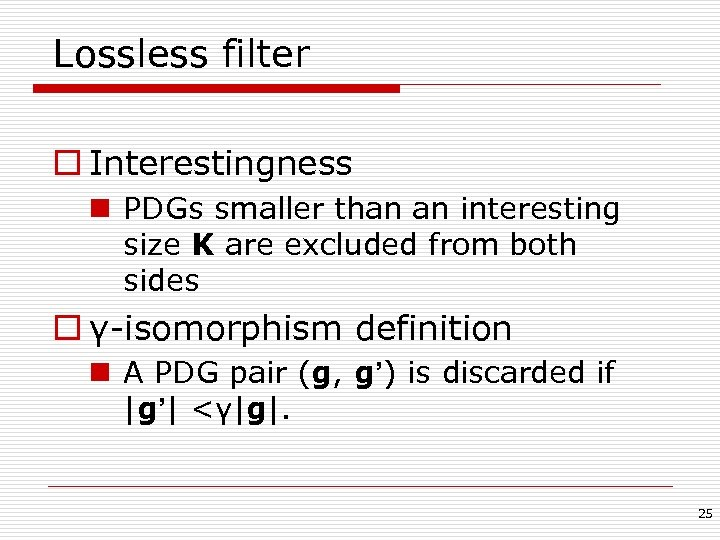Lossless filter o Interestingness n PDGs smaller than an interesting size K are excluded from both sides o γ-isomorphism definition n A PDG pair (g, g’) is discarded if |g’| <γ|g|. 25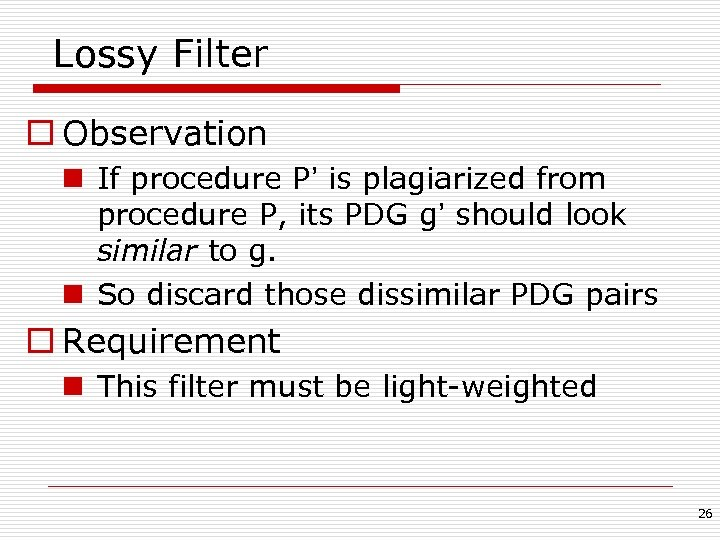Lossy Filter o Observation n If procedure P’ is plagiarized from procedure P, its PDG g’ should look similar to g. n So discard those dissimilar PDG pairs o Requirement n This filter must be light-weighted 26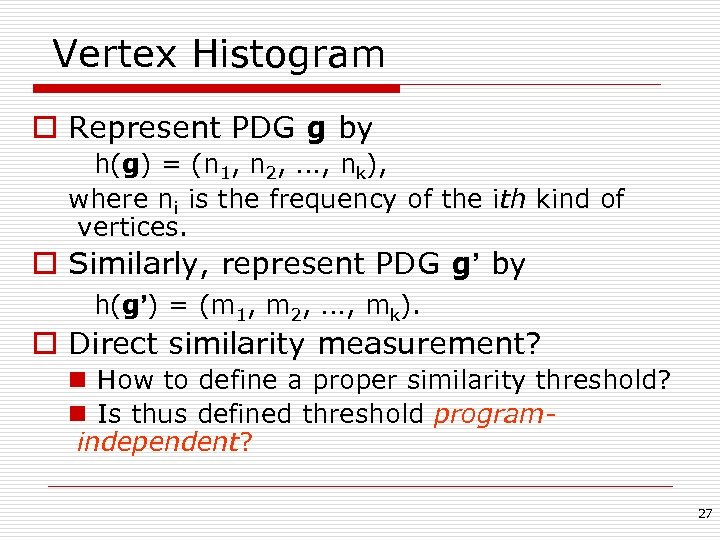Vertex Histogram o Represent PDG g by h(g) = (n 1, n 2, …, nk), where ni is the frequency of the ith kind of vertices. o Similarly, represent PDG g’ by h(g’) = (m 1, m 2, …, mk). o Direct similarity measurement? n How to define a proper similarity threshold? n Is thus defined threshold programindependent? 27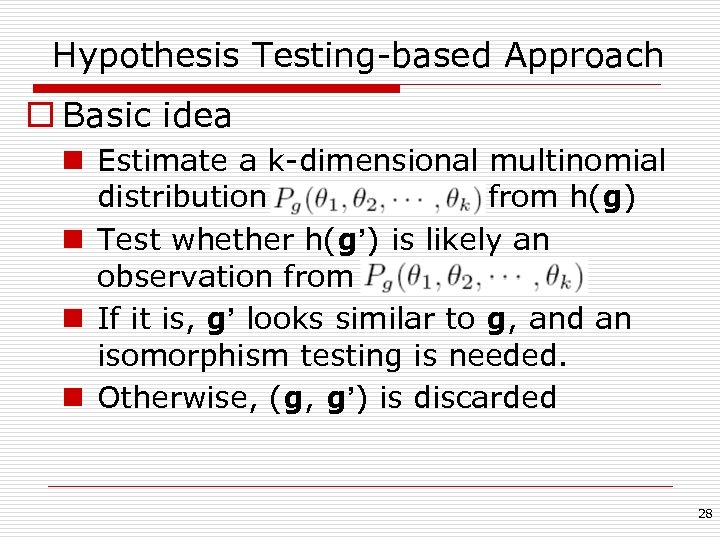Hypothesis Testing-based Approach o Basic idea n Estimate a k-dimensional multinomial distribution from h(g) n Test whether h(g’) is likely an observation from n If it is, g’ looks similar to g, and an isomorphism testing is needed. n Otherwise, (g, g’) is discarded 28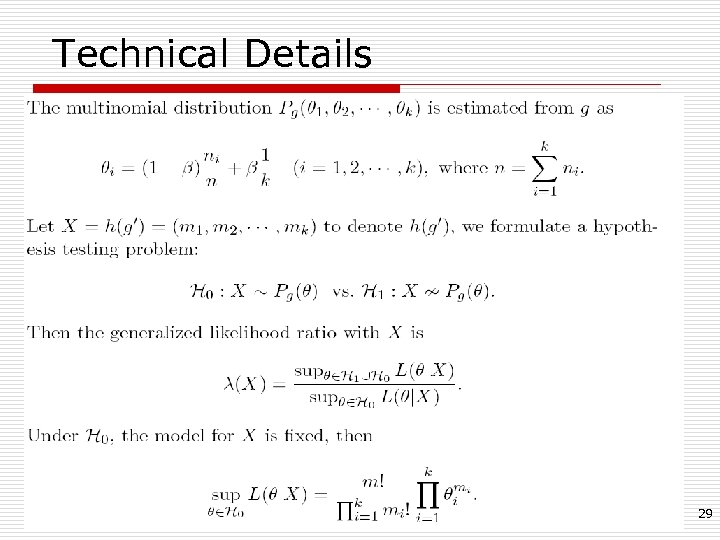Technical Details 29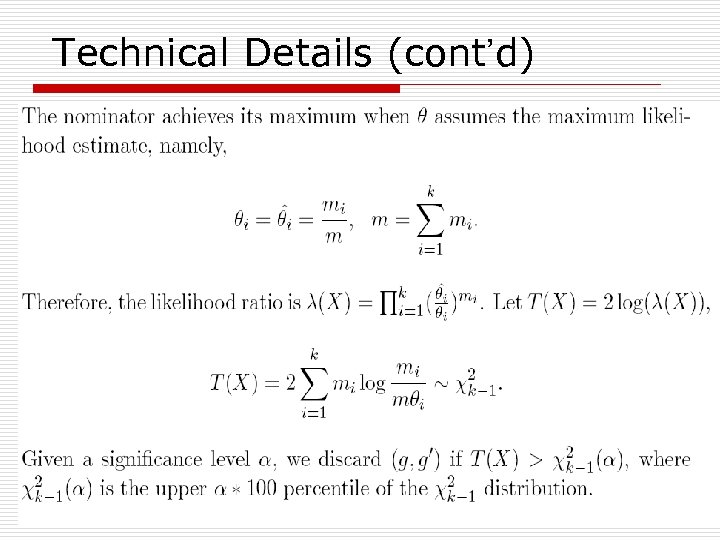Technical Details (cont’d) 30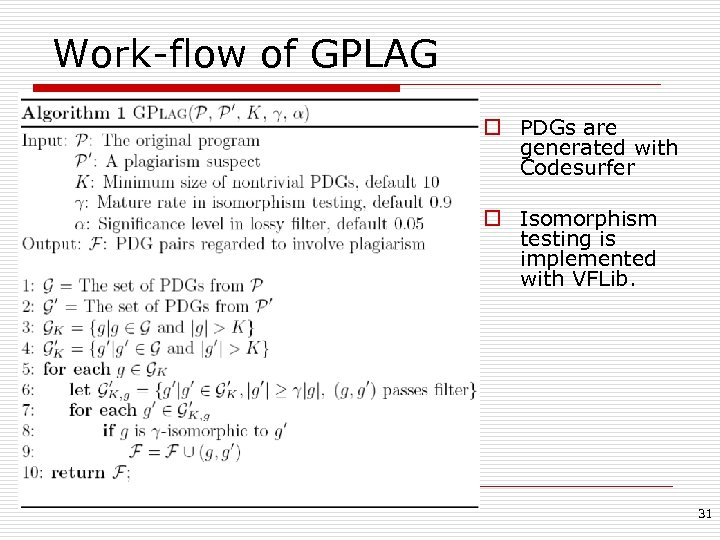Work-flow of GPLAG o PDGs are generated with Codesurfer o Isomorphism testing is implemented with VFLib. 31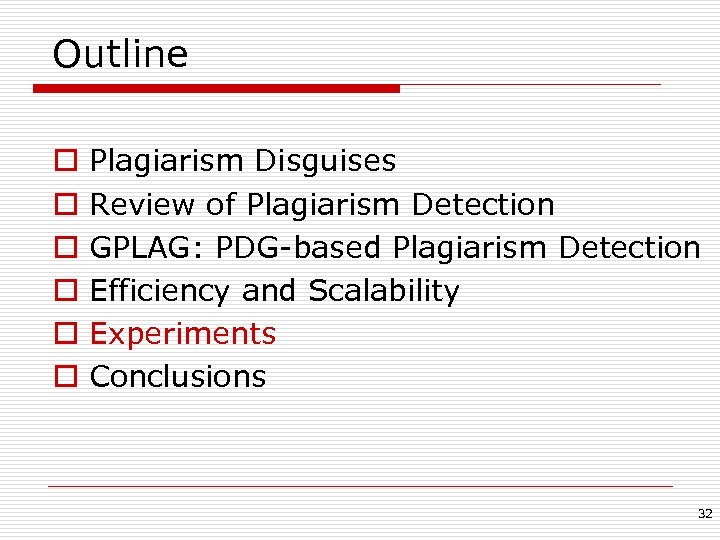Outline o o o Plagiarism Disguises Review of Plagiarism Detection GPLAG: PDG-based Plagiarism Detection Efficiency and Scalability Experiments Conclusions 32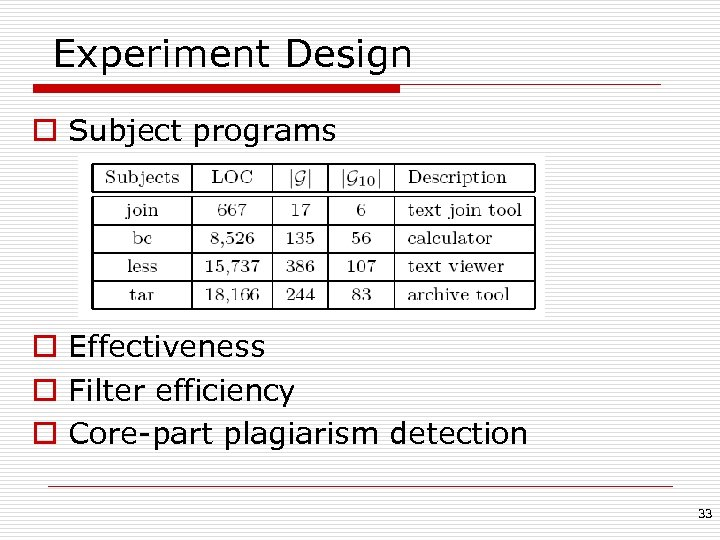Experiment Design o Subject programs o Effectiveness o Filter efficiency o Core-part plagiarism detection 33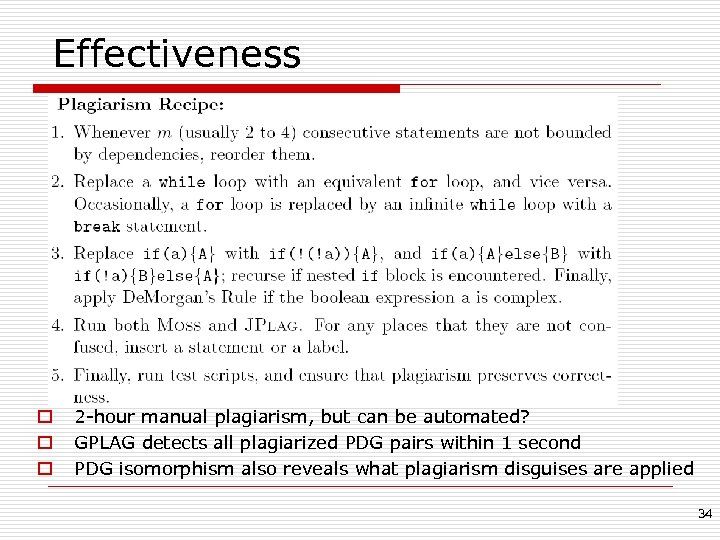Effectiveness o o o 2 -hour manual plagiarism, but can be automated? GPLAG detects all plagiarized PDG pairs within 1 second PDG isomorphism also reveals what plagiarism disguises are applied 34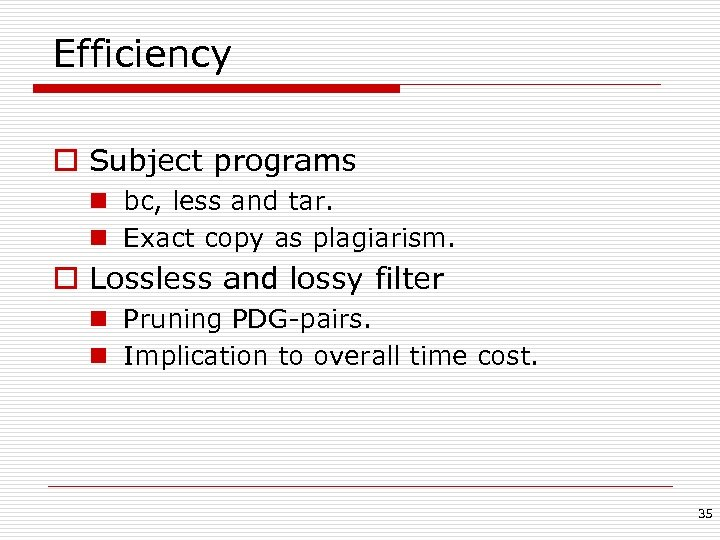Efficiency o Subject programs n bc, less and tar. n Exact copy as plagiarism. o Lossless and lossy filter n Pruning PDG-pairs. n Implication to overall time cost. 35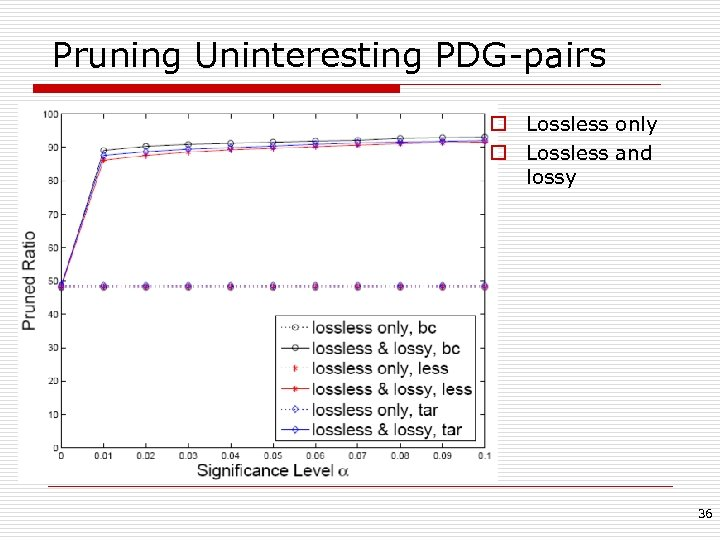Pruning Uninteresting PDG-pairs o Lossless only o Lossless and lossy 36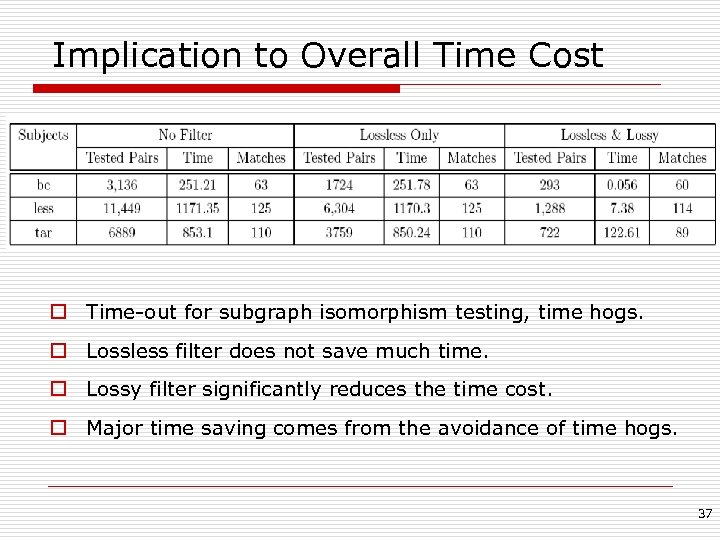Implication to Overall Time Cost o Time-out for subgraph isomorphism testing, time hogs. o Lossless filter does not save much time. o Lossy filter significantly reduces the time cost. o Major time saving comes from the avoidance of time hogs. 37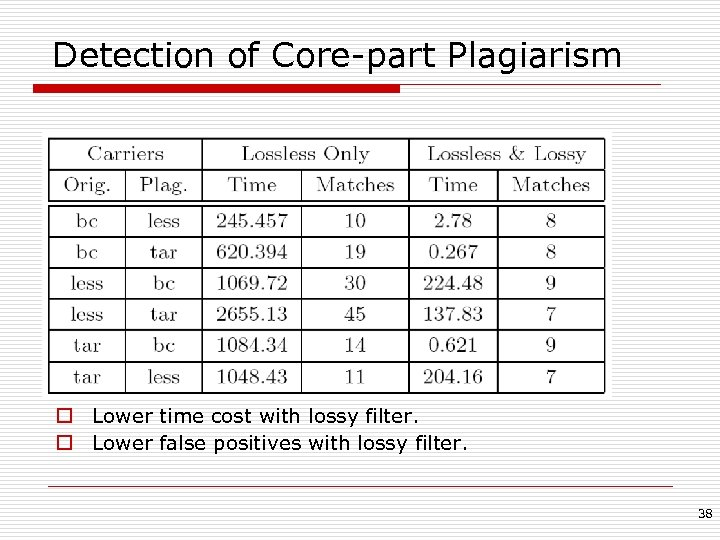Detection of Core-part Plagiarism o Lower time cost with lossy filter. o Lower false positives with lossy filter. 38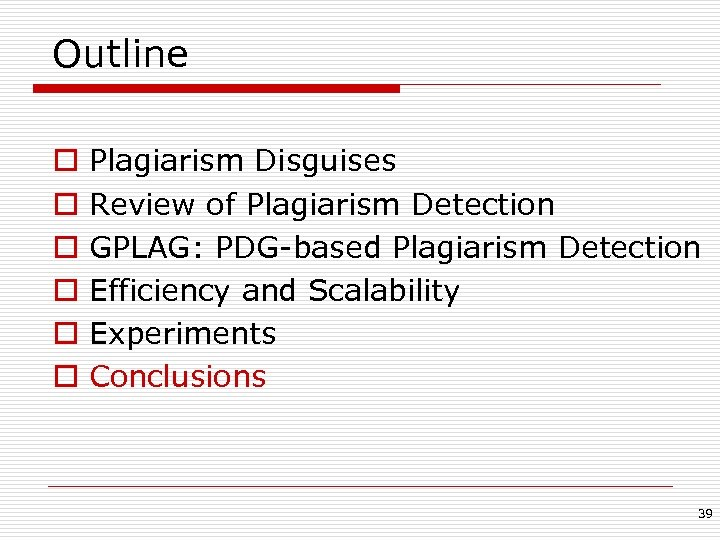Outline o o o Plagiarism Disguises Review of Plagiarism Detection GPLAG: PDG-based Plagiarism Detection Efficiency and Scalability Experiments Conclusions 39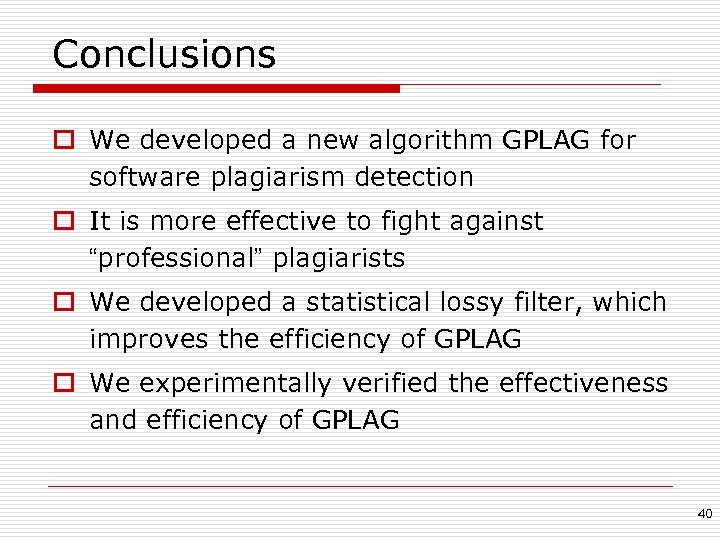Conclusions o We developed a new algorithm GPLAG for software plagiarism detection o It is more effective to fight against “professional” plagiarists o We developed a statistical lossy filter, which improves the efficiency of GPLAG o We experimentally verified the effectiveness and efficiency of GPLAG 40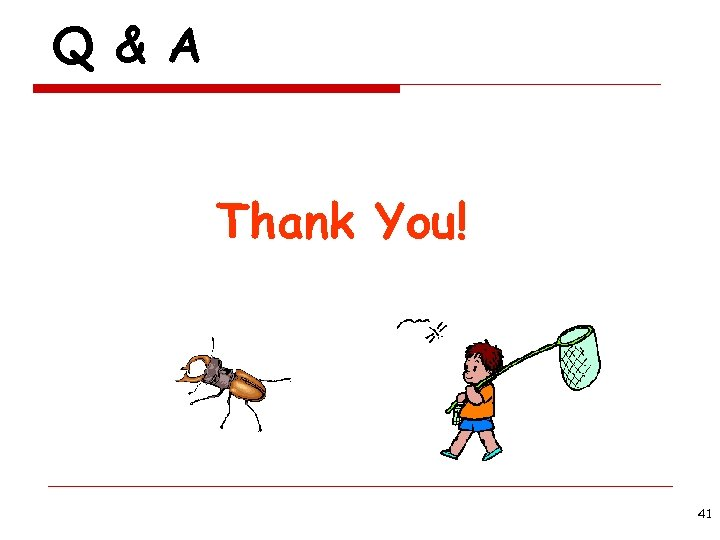Q & A Thank You! 41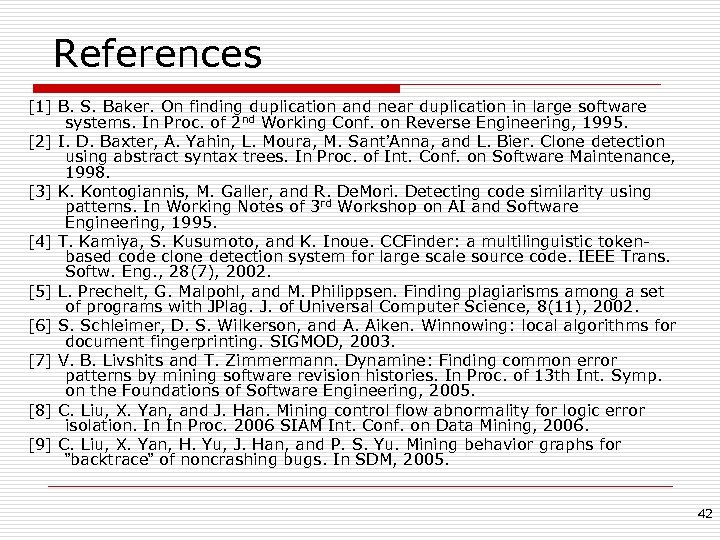References  B. S. Baker. On finding duplication and near duplication in large software systems. In Proc. of 2 nd Working Conf. on Reverse Engineering, 1995.  I. D. Baxter, A. Yahin, L. Moura, M. Sant’Anna, and L. Bier. Clone detection using abstract syntax trees. In Proc. of Int. Conf. on Software Maintenance, 1998.  K. Kontogiannis, M. Galler, and R. De. Mori. Detecting code similarity using patterns. In Working Notes of 3 rd Workshop on AI and Software Engineering, 1995.  T. Kamiya, S. Kusumoto, and K. Inoue. CCFinder: a multilinguistic tokenbased code clone detection system for large scale source code. IEEE Trans. Softw. Eng. , 28(7), 2002.  L. Prechelt, G. Malpohl, and M. Philippsen. Finding plagiarisms among a set of programs with JPlag. J. of Universal Computer Science, 8(11), 2002.  S. Schleimer, D. S. Wilkerson, and A. Aiken. Winnowing: local algorithms for document fingerprinting. SIGMOD, 2003.  V. B. Livshits and T. Zimmermann. Dynamine: Finding common error patterns by mining software revision histories. In Proc. of 13 th Int. Symp. on the Foundations of Software Engineering, 2005.  C. Liu, X. Yan, and J. Han. Mining control flow abnormality for logic error isolation. In In Proc. 2006 SIAM Int. Conf. on Data Mining, 2006.  C. Liu, X. Yan, H. Yu, J. Han, and P. S. Yu. Mining behavior graphs for ”backtrace” of noncrashing bugs. In SDM, 2005. 42